Related Articles
Inheritance in C++
• Difficulty Level : Easy
• Last Updated : 18 Mar, 2021

The capability of a class to derive properties and characteristics from another class is called Inheritance. Inheritance is one of the most important feature of Object Oriented Programming.
Sub Class: The class that inherits properties from another class is called Sub class or Derived Class.
Super Class:The class whose properties are inherited by sub class is called Base Class or Super class.
The article is divided into following subtopics:

Why and when to use inheritance?

Consider a group of vehicles. You need to create classes for Bus, Car and Truck. The methods fuelAmount(), capacity(), applyBrakes() will be same for all of the three classes. If we create these classes avoiding inheritance then we have to write all of these functions in each of the three classes as shown in below figure: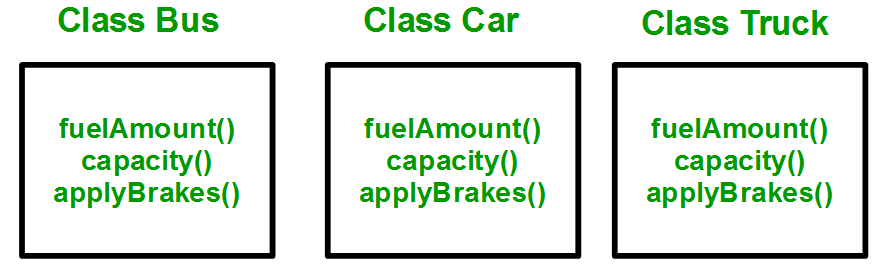You can clearly see that above process results in duplication of same code 3 times. This increases the chances of error and data redundancy. To avoid this type of situation, inheritance is used. If we create a class Vehicle and write these three functions in it and inherit the rest of the classes from the vehicle class, then we can simply avoid the duplication of data and increase re-usability. Look at the below diagram in which the three classes are inherited from vehicle class:Using inheritance, we have to write the functions only one time instead of three times as we have inherited rest of the three classes from base class(Vehicle).
Implementing inheritance in C++: For creating a sub-class which is inherited from the base class we have to follow the below syntax.
Syntax

```class subclass_name : access_mode base_class_name
{
//body of subclass
};```

Here, subclass_name is the name of the sub class, access_mode is the mode in which you want to inherit this sub class for example: public, private etc. and base_class_name is the name of the base class from which you want to inherit the sub class.
Note: A derived class doesn’t inherit access to private data members. However, it does inherit a full parent object, which contains any private members which that class declares.

## CPP

 `// C++ program to demonstrate implementation``// of Inheritance`` ` `#include ``using` `namespace` `std;` `//Base class``class` `Parent``{``    ``public``:``      ``int` `id_p;``};`` ` `// Sub class inheriting from Base Class(Parent)``class` `Child : ``public` `Parent``{``    ``public``:``      ``int` `id_c;``};` `//main function``int` `main()``   ``{``     ` `        ``Child obj1;``         ` `        ``// An object of class child has all data members``        ``// and member functions of class parent``        ``obj1.id_c = 7;``        ``obj1.id_p = 91;``        ``cout << ``"Child id is "` `<<  obj1.id_c << endl;``        ``cout << ``"Parent id is "` `<<  obj1.id_p << endl;``        ` `        ``return` `0;``   ``}`

Output:

```Child id is 7
Parent id is 91```

In the above program the ‘Child’ class is publicly inherited from the ‘Parent’ class so the public data members of the class ‘Parent’ will also be inherited by the class ‘Child’.
Modes of Inheritance

1. Public mode: If we derive a sub class from a public base class. Then the public member of the base class will become public in the derived class and protected members of the base class will become protected in derived class.
2. Protected mode: If we derive a sub class from a Protected base class. Then both public member and protected members of the base class will become protected in derived class.
3. Private mode: If we derive a sub class from a Private base class. Then both public member and protected members of the base class will become Private in derived class.

Note : The private members in the base class cannot be directly accessed in the derived class, while protected members can be directly accessed. For example, Classes B, C and D all contain the variables x, y and z in below example. It is just question of access.

## CPP

 `// C++ Implementation to show that a derived class``// doesn’t inherit access to private data members.``// However, it does inherit a full parent object``class` `A``{``public``:``    ``int` `x;``protected``:``    ``int` `y;``private``:``    ``int` `z;``};` `class` `B : ``public` `A``{``    ``// x is public``    ``// y is protected``    ``// z is not accessible from B``};` `class` `C : ``protected` `A``{``    ``// x is protected``    ``// y is protected``    ``// z is not accessible from C``};` `class` `D : ``private` `A    ``// 'private' is default for classes``{``    ``// x is private``    ``// y is private``    ``// z is not accessible from D``};`

The below table summarizes the above three modes and shows the access specifier of the members of base class in the sub class when derived in public, protected and private modes: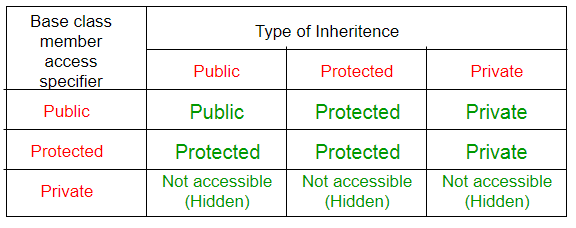Types of Inheritance in C++

1. Single Inheritance: In single inheritance, a class is allowed to inherit from only one class. i.e. one sub class is inherited by one base class only.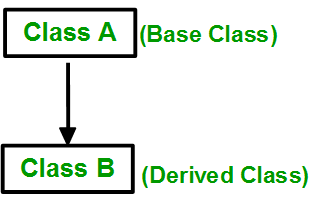Syntax

```class subclass_name : access_mode base_class
{
//body of subclass
};```

## CPP

 `// C++ program to explain``// Single inheritance``#include ``using` `namespace` `std;` `// base class``class` `Vehicle {``  ``public``:``    ``Vehicle()``    ``{``      ``cout << ``"This is a Vehicle"` `<< endl;``    ``}``};` `// sub class derived from a single base classes``class` `Car: ``public` `Vehicle{` `};` `// main function``int` `main()``{  ``    ``// creating object of sub class will``    ``// invoke the constructor of base classes``    ``Car obj;``    ``return` `0;``}`

Output:

`This is a vehicle`

2. Multiple Inheritance: Multiple Inheritance is a feature of C++ where a class can inherit from more than one classes. i.e one sub class is inherited from more than one base classes.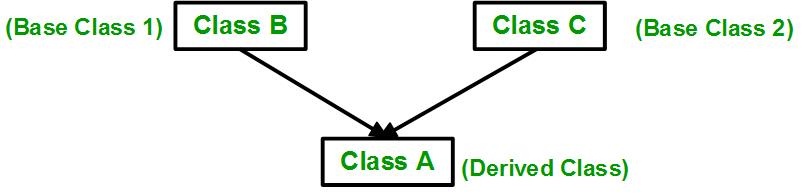Syntax

```class subclass_name : access_mode base_class1, access_mode base_class2, ....
{
//body of subclass
};```

Here, the number of base classes will be separated by a comma (‘, ‘) and access mode for every base class must be specified.

## CPP

 `// C++ program to explain``// multiple inheritance``#include ``using` `namespace` `std;` `// first base class``class` `Vehicle {``  ``public``:``    ``Vehicle()``    ``{``      ``cout << ``"This is a Vehicle"` `<< endl;``    ``}``};` `// second base class``class` `FourWheeler {``  ``public``:``    ``FourWheeler()``    ``{``      ``cout << ``"This is a 4 wheeler Vehicle"` `<< endl;``    ``}``};` `// sub class derived from two base classes``class` `Car: ``public` `Vehicle, ``public` `FourWheeler {` `};` `// main function``int` `main()``{  ``    ``// creating object of sub class will``    ``// invoke the constructor of base classes``    ``Car obj;``    ``return` `0;``}`

Output:

```This is a Vehicle
This is a 4 wheeler Vehicle```

Please visit this link to learn multiple inheritance in details.
3. Multilevel Inheritance: In this type of inheritance, a derived class is created from another derived class.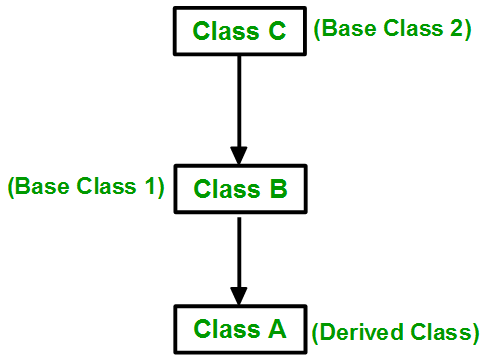## CPP

 `// C++ program to implement``// Multilevel Inheritance``#include ``using` `namespace` `std;` `// base class``class` `Vehicle``{``  ``public``:``    ``Vehicle()``    ``{``      ``cout << ``"This is a Vehicle"` `<< endl;``    ``}``};` `// first sub_class derived from class vehicle``class` `fourWheeler: ``public` `Vehicle``{  ``public``:``    ``fourWheeler()``    ``{``      ``cout<<``"Objects with 4 wheels are vehicles"``<

Output:

```This is a Vehicle
Objects with 4 wheels are vehicles
Car has 4 Wheels```

4. Hierarchical Inheritance: In this type of inheritance, more than one sub class is inherited from a single base class. i.e. more than one derived class is created from a single base class.## CPP

 `// C++ program to implement``// Hierarchical Inheritance``#include ``using` `namespace` `std;` `// base class``class` `Vehicle``{``  ``public``:``    ``Vehicle()``    ``{``      ``cout << ``"This is a Vehicle"` `<< endl;``    ``}``};`  `// first sub class``class` `Car: ``public` `Vehicle``{` `};` `// second sub class``class` `Bus: ``public` `Vehicle``{``    ` `};` `// main function``int` `main()``{  ``    ``// creating object of sub class will``    ``// invoke the constructor of base class``    ``Car obj1;``    ``Bus obj2;``    ``return` `0;``}`

Output:

```This is a Vehicle
This is a Vehicle```

5. Hybrid (Virtual) Inheritance: Hybrid Inheritance is implemented by combining more than one type of inheritance. For example: Combining Hierarchical inheritance and Multiple Inheritance.
Below image shows the combination of hierarchical and multiple inheritance: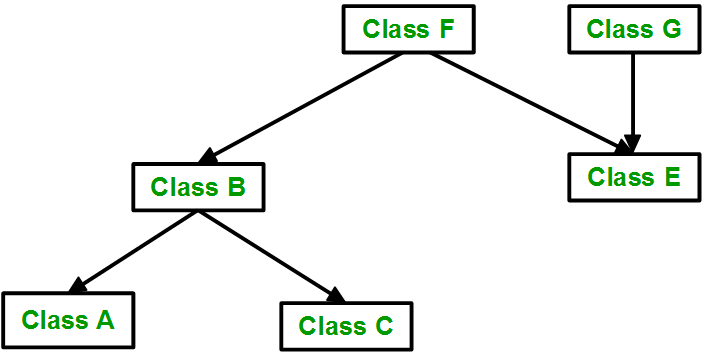## CPP

 `// C++ program for Hybrid Inheritance` `#include ``using` `namespace` `std;` `// base class``class` `Vehicle``{``  ``public``:``    ``Vehicle()``    ``{``      ``cout << ``"This is a Vehicle"` `<< endl;``    ``}``};` `//base class``class` `Fare``{``    ``public``:``    ``Fare()``    ``{``        ``cout<<``"Fare of Vehicle\n"``;``    ``}``};` `// first sub class``class` `Car: ``public` `Vehicle``{` `};` `// second sub class``class` `Bus: ``public` `Vehicle, ``public` `Fare``{``    ` `};` `// main function``int` `main()``{  ``    ``// creating object of sub class will``    ``// invoke the constructor of base class``    ``Bus obj2;``    ``return` `0;``}`

Output:

```This is a Vehicle
Fare of Vehicle```

6. A special case of hybrid inheritance : Multipath inheritance
A derived class with two base classes and these two base classes have one common base class is called multipath inheritance. An ambiguity can arrise in this type of inheritance.Consider the following program:

## CPP

 `// C++ program demonstrating ambiguity in Multipath``// Inheritance` `#include ``#include ``class` `ClassA {``public``:``    ``int` `a;``};` `class` `ClassB : ``public` `ClassA {``public``:``    ``int` `b;``};``class` `ClassC : ``public` `ClassA {``public``:``    ``int` `c;``};` `class` `ClassD : ``public` `ClassB, ``public` `ClassC {``public``:``    ``int` `d;``};` `void` `main()``{` `    ``ClassD obj;` `    ``// obj.a = 10;                   //Statement 1, Error``    ``// obj.a = 100;                 //Statement 2, Error` `    ``obj.ClassB::a = 10; ``// Statement 3``    ``obj.ClassC::a = 100; ``// Statement 4` `    ``obj.b = 20;``    ``obj.c = 30;``    ``obj.d = 40;` `    ``cout << ``"\n A from ClassB  : "` `<< obj.ClassB::a;``    ``cout << ``"\n A from ClassC  : "` `<< obj.ClassC::a;` `    ``cout << ``"\n B : "` `<< obj.b;``    ``cout << ``"\n C : "` `<< obj.c;``    ``cout << ``"\n D : "` `<< obj.d;``}`

Output:

```A from ClassB  : 10
A from ClassC  : 100
B : 20
C : 30
D : 40```

In the above example, both ClassB & ClassC inherit ClassA, they both have single copy of ClassA. However ClassD inherit both ClassB & ClassC, therefore ClassD have two copies of ClassA, one from ClassB and another from ClassC.
If we need to access the data member a of ClassA through the object of ClassD, we must specify the path from which a will be accessed, whether it is from ClassB or ClassC, bco’z compiler can’t differentiate between two copies of ClassA in ClassD.
There are 2 ways to avoid this ambiguity:
Avoiding ambiguity using scope resolution operator:
Using scope resolution operator we can manually specify the path from which data member a will be accessed, as shown in statement 3 and 4, in the above example.

## CPP

 `obj.ClassB::a = 10;        ``//Statement 3``obj.ClassC::a = 100;      ``//Statement 4`

Note : Still, there are two copies of ClassA in ClassD.
Avoiding ambiguity using virtual base class:

## CPP

 `#include``     ``#include` `     ``class` `ClassA``     ``{``            ``public``:``            ``int` `a;``     ``};` `     ``class` `ClassB : ``virtual` `public` `ClassA``     ``{``            ``public``:``            ``int` `b;``     ``};``     ``class` `ClassC : ``virtual` `public` `ClassA``     ``{``            ``public``:``            ``int` `c;``     ``};` `     ``class` `ClassD : ``public` `ClassB, ``public` `ClassC``     ``{``            ``public``:``            ``int` `d;``     ``};` `     ``void` `main()``     ``{` `            ``ClassD obj;` `            ``obj.a = 10;        ``//Statement 3``            ``obj.a = 100;      ``//Statement 4` `            ``obj.b = 20;``            ``obj.c = 30;``            ``obj.d = 40;` `            ``cout<< ``"\n A : "``<< obj.a;``            ``cout<< ``"\n B : "``<< obj.b;``            ``cout<< ``"\n C : "``<< obj.c;``            ``cout<< ``"\n D : "``<< obj.d;` `     ``}`

Output:

```A : 100
B : 20
C : 30
D : 40```

According to the above example, ClassD has only one copy of ClassA, therefore, statement 4 will overwrite the value of a, given at statement 3.
This article is contributed by Harsh Agarwal. If you like GeeksforGeeks and would like to contribute, you can also write an article using contribute.geeksforgeeks.org or mail your article to contribute@geeksforgeeks.org. See your article appearing on the GeeksforGeeks main page and help other Geeks.

Want to learn from the best curated videos and practice problems, check out the C++ Foundation Course for Basic to Advanced C++ and C++ STL Course for foundation plus STL.  To complete your preparation from learning a language to DS Algo and many more,  please refer Complete Interview Preparation Course.
My Personal Notes arrow_drop_up